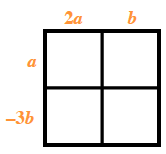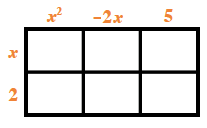### Home > CCA > Chapter 6 > Lesson 6.2.3 > Problem6-88

6-88.

Multiply each pair of polynomials. Homework Help ✎

1. $(2a+b)(a−3b)$

Use a generic rectangle to multiply the polynomials.1. $( x + 2 ) ( x ^ { 2 } - 2 x + 5 )$